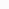# Round up a number to highest 10s place

0

Recently we faced a problem that we need to round-up a number to greatest 10s place.
For ex: we needed to round-up a number as follows
1023 -> 2000
37911 -> 40000
912345 –> 1000000

First we checked Math.Round(). However, it did not solve our problem.  Math.Round() supports only rounds a value to the nearest integer or the specified number of fractional digits. Further checking we come up a solution by using Math.Ceiling() method.

Math.Ceiling()returns the smallest integral value that is greater than or equal to the specified double-precision floating point number.
For ex:
Math.Ceiling(7.3)     -> 8
Math.Ceiling(7.64)     -> 8
Math.Ceiling(0.12)     -> 1

Below is the solution we came up

```decimal[] values = { 1023, 37911, 23000, 1234, 912345 };
foreach (var value in values) {
//// get the lenght of the 10s places;
var length = (int)Math.Log10((double)value);
//// using Math.Pow() calculate the power of 10s
var power = Math.Pow(10, length);
//// var roundadValue = Math.Ceiling(1023 / 1000) * 1000;
var roundadValue = Math.Ceiling(value / (decimal)power) * (decimal)power;

}
```## The Logic

```
Math.Ceiling(value / (decimal)power) * (decimal)power;
var roundadValue = Math.Ceiling(1023 / 1000) * 1000;
```

So, for any number, fist compute power of 10s and then it use for the above calculation to get the results.